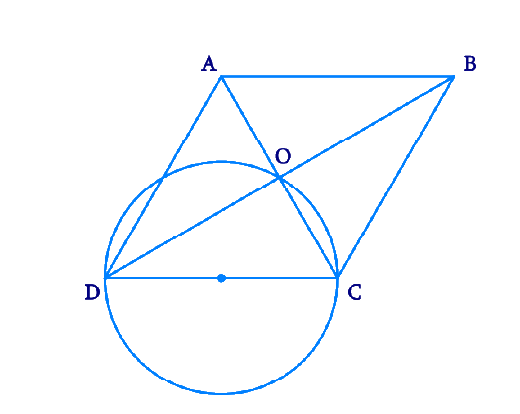# Ex.10.6 Q5 Circles Solution - NCERT Maths Class 9

Go back to  'Ex.10.6'

## Question

Prove that the circle drawn with any side of a rhombus as diameter, passes through the point of intersection of its diagonals.

Video Solution
Circles
Ex 10.6 | Question 5

## Text Solution

What is known?

Diameter of the circle is the length of one side of rhombus.

What is unknown?

Proof of  the circle passes through the point of intersection of rhombus diagonals.

Reasoning:

The diagonals of rhombus intersect at \begin{align}90^\circ.\end{align}

Steps:Let \begin{align}{ABCD}\end{align} be a rhombus in which diagonals are intersecting at point \begin{align}{O}\end{align} and a circle is drawn while taking side \begin{align}{CD}\end{align} as its diameter. We know that a diameter subtends \begin{align}90^\circ\end{align} on the arc.

\begin{align}∴{COD }= 90^\circ\end{align}

Also, in rhombus, the diagonals intersect each other at \begin{align}90^\circ.\end{align}

\begin {align} \angle {AOB} & =\angle {BOC}\\ &=\angle {COD}\\ & =\angle {DOA} \\ & =90^{\circ}\end {align}

Clearly, point \begin {align}{O} \end {align} has to lie on the circle.

Video Solution
Circles
Ex 10.6 | Question 5

Learn from the best math teachers and top your exams

• Live one on one classroom and doubt clearing
• Practice worksheets in and after class for conceptual clarity
• Personalized curriculum to keep up with school